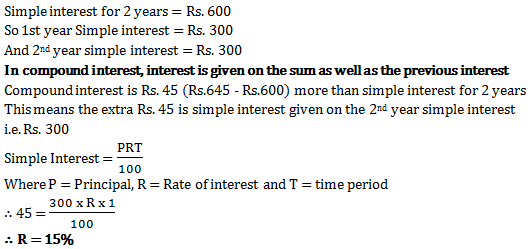# Simple Interest - Quantitative Aptitude (MCQ) questions for Q. 29336

Q.  If simple interest for 2 years for a sum is Rs. 600 and compound interest for the same sum for 2 years and same rate of interest is Rs. 645, what will be the rate of interest?
- Published on 11 Apr 17

a. 10%
b. 15%
c. 30%
d. 5%